# Korovkin second theorem via statistical summability $\left(C,1\right)$

## Abstract

Korovkin type approximation theorems are useful tools to check whether a given sequence ${\left({L}_{n}\right)}_{n\ge 1}$ of positive linear operators on $C\left[0,1\right]$ of all continuous functions on the real interval $\left[0,1\right]$ is an approximation process. That is, these theorems exhibit a variety of test functions, which assure that the approximation property holds on the whole space if it holds for them. Such a property was discovered by Korovkin in 1953 for the functions 1, x and ${x}^{2}$ in the space $C\left[0,1\right]$ as well as for the functions 1, cos and sin in the space of all continuous 2π-periodic functions on the real line. In this paper, we use the notion of statistical summability $\left(C,1\right)$ to prove the Korovkin approximation theorem for the functions 1, cos and sin in the space of all continuous 2π-periodic functions on the real line and show that our result is stronger. We also study the rate of weighted statistical convergence.

MSC:41A10, 41A25, 41A36, 40A30, 40G15.

## 1 Introduction and preliminaries

We shall denote by be the set of all natural numbers. Let $K\subseteq \mathbb{N}$ and ${K}_{n}=\left\{k\le n:k\in K\right\}$. Then the natural density of K is defined by $\delta \left(K\right)={lim}_{n}{n}^{-1}|{K}_{n}|$ if the limit exists, where the vertical bars indicate the number of elements in the enclosed set. The sequence $x=\left({x}_{k}\right)$ is said to be statistically convergent to L if for every $\epsilon >0$, the set ${K}_{\epsilon }:=\left\{k\in \mathbb{N}:|{x}_{k}-L|\ge \epsilon \right\}$ has natural density zero (cf. [1, 2]), i.e. for each $\epsilon >0$,

$\underset{n}{lim}\frac{1}{n}|\left\{j\le n:|{x}_{j}-L|\ge \epsilon \right\}|=0.$

In this case, we write $L=st\text{-}limx$. Note that every convergent sequence is statistically convergent but not conversely.

Recently, Móricz  has defined the concept of statistical summability $\left(C,1\right)$ as follows:

For a sequence $x=\left({x}_{k}\right)$, let us write ${t}_{n}=\frac{1}{n+1}{\sum }_{k=0}^{n}{x}_{k}$. We say that a sequence $x=\left({x}_{k}\right)$ is statistiallly summable $\left(C,1\right)$ if $st\text{-}{lim}_{n\to \mathrm{\infty }}{t}_{n}=L$. In this case we write $L={C}_{1}\left(st\right)\text{-}limx$.

Note that evey $\left(C,1\right)$-summable sequence is also statistiallly summable $\left(C,1\right)$ to the same limit but not conversely.

Let $x=\left({x}_{k}\right)$ be a sequence defined by

(1.1)

Then this sequence is neither convergent nor statistically convergent. But x is $\left(C,1\right)$-summable to 0, and hence statistiallly summable $\left(C,1\right)$ to 0.

For more details, related concepts and applications, we refer to  and references therein.

Let $F\left(\mathbb{R}\right)$ denote the linear space of all real-valued functions defined on . Let $C\left(\mathbb{R}\right)$ be the space of all bounded and continuous functions f defined on . We know that $C\left(\mathbb{R}\right)$ is a Banach space with norm

${\parallel f\parallel }_{\mathrm{\infty }}:=\underset{x\in \mathbb{R}}{sup}|f\left(x\right)|,\phantom{\rule{1em}{0ex}}f\in C\left(\mathbb{R}\right).$

We denote by ${C}_{2\pi }\left(\mathbb{R}\right)$ the space of all 2π-periodic functions $f\in C\left(\mathbb{R}\right)$, which is a Banach spaces with

${\parallel f\parallel }_{2\pi }=\underset{t\in \mathbb{R}}{sup}|f\left(t\right)|.$

The classical Korovkin first and second theorems state as follows [18, 19].

Theorem I Let $\left({T}_{n}\right)$ be a sequence of positive linear operators from $C\left[0,1\right]$ into $F\left[0,1\right]$. Then ${lim}_{n}{\parallel {T}_{n}\left(f,x\right)-f\left(x\right)\parallel }_{\mathrm{\infty }}=0$, for all $f\in C\left[0,1\right]$ if and only if ${lim}_{n}{\parallel {T}_{n}\left({f}_{i},x\right)-{f}_{i}\left(x\right)\parallel }_{\mathrm{\infty }}=0$, for $i=0,1,2$, where ${f}_{0}\left(x\right)=1$, ${f}_{1}\left(x\right)=x$ and ${f}_{2}\left(x\right)={x}^{2}$.

Theorem II Let $\left({T}_{n}\right)$ be a sequence of positive linear operators from ${C}_{2\pi }\left(\mathbb{R}\right)$ into $F\left(\mathbb{R}\right)$. Then ${lim}_{n}{\parallel {T}_{n}\left(f,x\right)-f\left(x\right)\parallel }_{\mathrm{\infty }}=0$, for all $f\in {C}_{2\pi }\left(\mathbb{R}\right)$ if and only if ${lim}_{n}{\parallel {T}_{n}\left({f}_{i},x\right)-{f}_{i}\left(x\right)\parallel }_{\mathrm{\infty }}=0$, for $i=0,1,2$, where ${f}_{0}\left(x\right)=1$, ${f}_{1}\left(x\right)=cosx$ and ${f}_{2}\left(x\right)=sinx$.

Several mathematicians have worked on extending or generalizing the Korovkin’s theorems in many ways and to several settings, including function spaces, abstract Banach lattices, Banach algebras, Banach spaces and so on. This theory is very useful in real analysis, functional analysis, harmonic analysis, measure theory, probability theory, summability theory and partial differential equations. But the foremost applications are concerned with constructive approximation theory, which uses it as a valuable tool. Even today, the development of Korovkin-type approximation theory is far from complete. Note that the first and the second theorems of Korovkin are actually equivalent to the algebraic and the trigonometric version, respectively, of the classical Weierstrass approximation theorem . Recently, the Korovkin second theorem is proved in  by using the concept of statistical convergence. Quite recently, Korovkin second theorem is proved by Demirci and Dirik  for statistical σ-convergence . For some recent work on this topic, we refer to . In this work, we prove Korovkin second theorem by applying the notion of statistical summability $\left(C,1\right)$. We also give an example to justify that our result is stronger than Theorem II.

## 2 Main result

We write ${L}_{n}\left(f;x\right)$ for ${L}_{n}\left(f\left(s\right);x\right)$; and we say that L is a positive operator if $L\left(f;x\right)\ge 0$ for all $f\left(x\right)\ge 0$.

Theorem 2.1 Let $\left({T}_{k}\right)$ be a sequence of positive linear operators from ${C}_{2\pi }\left(\mathbb{R}\right)$ into ${C}_{2\pi }\left(\mathbb{R}\right)$. Then for all $f\in {C}_{2\pi }\left(\mathbb{R}\right)$

${C}_{1}\left(st\right)\text{-}\underset{k\to \mathrm{\infty }}{lim}{\parallel {T}_{k}\left(f;x\right)-f\left(x\right)\parallel }_{2\pi }=0.$
(2.1)

if and only if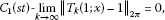(2.2)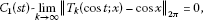(2.3)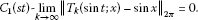(2.4)

Proof Since each of 1, cosx, sinx belongs to ${C}_{2\pi }\left(\mathbb{R}\right)$, conditions (2.2)-(2.4) follow immediately from (2.1). Let the conditions (2.2)-(2.4) hold and $f\in {C}_{2\pi }\left(\mathbb{R}\right)$. Let I be a closed subinterval of length 2π of . Fix $x\in I$. By the continuity of f at x, it follows that for given $\epsilon >0$ there is a number $\delta >0$ such that for all t

$|f\left(t\right)-f\left(x\right)|<\epsilon ,$
(2.5)

whenever $|t-x|<\delta$. Since f is bounded, it follows that

$|f\left(t\right)-f\left(x\right)|\le {\parallel f\parallel }_{2\pi },$
(2.6)

for all $t\in \mathbb{R}$. For all $t\in \left(x-\delta ,2\pi +x-\delta \right]$, it is well known that

$|f\left(t\right)-f\left(x\right)|<\epsilon +\frac{2{\parallel f\parallel }_{2\pi }}{{sin}^{2}\frac{\delta }{2}}\psi \left(t\right),$
(2.7)

where $\psi \left(t\right)={sin}^{2}\left(\frac{t-x}{2}\right)$. Since the function $f\in {C}_{2\pi }\left(\mathbb{R}\right)$ is 2π-periodic, the inequality (2.6) holds for $t\in \mathbb{R}$. We can also write (2.7) as

$-\epsilon -\frac{2{\parallel f\parallel }_{2\pi }}{{sin}^{2}\frac{\delta }{2}}\psi \left(t\right)

Now applying the operator ${T}_{k}\left(1;x\right)$ to this inequality, we obtain

$\begin{array}{rcl}{T}_{k}\left(1;x\right)\left(-\epsilon -\frac{2{\parallel f\parallel }_{2\pi }}{{sin}^{2}\frac{\delta }{2}}\psi \left(t\right)\right)& <& {T}_{k}\left(1;x\right)\left(f\left(t\right)-f\left(x\right)\right)\\ <& {T}_{k}\left(1;x\right)\left(\epsilon +\frac{2{\parallel f\parallel }_{2\pi }}{{sin}^{2}\frac{\delta }{2}}\psi \left(t\right)\right).\end{array}$

As we know that x is fixed and so $f\left(x\right)$ is a constant number. Therefore,

$\begin{array}{rcl}-\epsilon {T}_{k}\left(1;x\right)-\frac{2{\parallel f\parallel }_{2\pi }}{{sin}^{2}\frac{\delta }{2}}{T}_{k}\left(\psi \left(t\right);x\right)& <& {T}_{k}\left(f;x\right)-f\left(x\right){T}_{k}\left(1;x\right)\\ <& \epsilon {T}_{k}\left(1;x\right)+\frac{2{\parallel f\parallel }_{2\pi }}{{sin}^{2}\frac{\delta }{2}}{T}_{k}\left(\psi \left(t\right);x\right).\end{array}$
(2.8)

Also,

$\begin{array}{rcl}{T}_{k}\left(f;x\right)-f\left(x\right)& =& {T}_{k}\left(f;x\right)-f\left(x\right){T}_{k}\left(1;x\right)+f\left(x\right){T}_{k}\left(1;x\right)-f\left(x\right)\\ =& {T}_{k}\left(f;x\right)-f\left(x\right){T}_{k}\left(1;x\right)+f\left(x\right)\left[{T}_{k}\left(1;x\right)-1\right].\end{array}$
(2.9)

From (2.8) and (2.9), we have

${T}_{k}\left(f;x\right)-f\left(x\right)<\epsilon {T}_{k}\left(1;x\right)+\frac{2{\parallel f\parallel }_{2\pi }}{{sin}^{2}\frac{\delta }{2}}{T}_{k}\left(\psi \left(t\right);x\right)+f\left(x\right)\left[{T}_{k}\left(1;x\right)-1\right].$
(2.10)

Now

$\begin{array}{rcl}{T}_{k}\left(\psi \left(t\right);x\right)& =& {T}_{k}\left({sin}^{2}\left(\frac{t-x}{2}\right);x\right)\\ =& {T}_{k}\left(\frac{1}{2}\left(1-costcosx-sintsinx\right);x\right)\\ =& \frac{1}{2}\left\{{T}_{k}\left(1;x\right)-cosx{T}_{k}\left(cost;x\right)-sinx{T}_{k}\left(sint;x\right)\right\}\\ =& \frac{1}{2}\left\{\left[{T}_{k}\left(1;x\right)-1\right]-cosx\left[{T}_{k}\left(cost;x\right)-cosx\right]-sinx\left[{T}_{k}\left(sint;x\right)-sinx\right]\right\}.\end{array}$

Substituting the value of ${T}_{k}\left(\psi \left(t\right);x\right)$ in (2.10), we get

$\begin{array}{rcl}{T}_{k}\left(f;x\right)-f\left(x\right)& <& \epsilon {T}_{k}\left(1;x\right)+\frac{{\parallel f\parallel }_{2\pi }}{{sin}^{2}\frac{\delta }{2}}\left\{\left[{T}_{k}\left(1;x\right)-1\right]-cosx\left[{T}_{k}\left(cost;x\right)-cosx\right]\\ -sinx\left[{T}_{k}\left(sint;x\right)-sinx\right]\right\}+f\left(x\right)\left[{T}_{k}\left(1;x\right)-1\right]\\ =& \epsilon +\epsilon \left[{T}_{k}\left(1;x\right)-1\right]+\frac{{\parallel f\parallel }_{2\pi }}{{sin}^{2}\frac{\delta }{2}}\left\{\left[{T}_{k}\left(1;x\right)-1\right]-cosx\left[{T}_{k}\left(cost;x\right)-cosx\right]\\ -sinx\left[{T}_{k}\left(sint;x\right)-sinx\right]\right\}+f\left(x\right)\left[{T}_{k}\left(1;x\right)-1\right].\end{array}$

Therefore,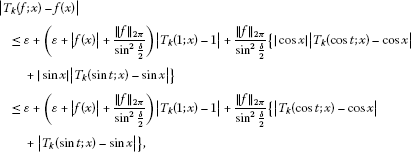since $|cosx|\le 1$ and $|sinx|\le 1$ for all $x\in I$. Now taking ${sup}_{x\in I}$, we get

$\begin{array}{rcl}{\parallel {T}_{k}\left(f;x\right)-f\left(x\right)\parallel }_{2\pi }& \le & \epsilon +K\left({\parallel {T}_{k}\left(1;x\right)-1\parallel }_{2\pi }+{\parallel {T}_{k}\left(cost;x\right)-cosx\parallel }_{2\pi }\\ +{\parallel {T}_{k}\left(sint;x\right)-sinx\parallel }_{2\pi }\right),\end{array}$

where

$K=max\left\{\epsilon +{\parallel f\parallel }_{2\pi }+\frac{{\parallel f\parallel }_{2\pi }}{{sin}^{2}\frac{\delta }{2}},\frac{{\parallel f\parallel }_{2\pi }}{{sin}^{2}\frac{\delta }{2}}\right\}.$

Now replacing ${T}_{k}\left(\cdot ;x\right)$ by $\frac{1}{n+1}{\sum }_{k=0}^{n}{T}_{k}\left(\cdot ;x\right)={L}_{n}\left(\cdot ;x\right)$

$\begin{array}{rcl}{\parallel {L}_{n}\left(f;x\right)-f\left(x\right)\parallel }_{\mathrm{\infty }}& \le & \epsilon +K\left({\parallel {L}_{n}\left(1;x\right)-1\parallel }_{2\pi }+{\parallel {L}_{n}\left(cost;x\right)-cosx\parallel }_{2\pi }\\ +{\parallel {L}_{n}\left(sint;x\right)-sinx\parallel }_{2\pi }\right).\end{array}$
(2.11)

For a given $r>0$, choose ${\epsilon }^{\mathrm{\prime }}>0$ such that ${\epsilon }^{\mathrm{\prime }}. Define the following sets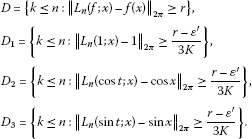Then

$D\subset {D}_{1}\cup {D}_{2}\cup {D}_{3},$

and so

$\delta \left(D\right)\le \delta \left({D}_{1}\right)+\delta \left({D}_{2}\right)+\delta \left({D}_{3}\right).$

Therefore, using conditions (2.1), (2.2) and (2.3), we get

${C}_{1}\left(st\right)\text{-}\underset{n}{lim}{\parallel {T}_{n}\left(f,x\right)-f\left(x\right)\parallel }_{2\pi }=0.$

This completes the proof of the theorem. □

## 3 Rate of weighted statistical convergence

In this section, we study the rate of weighted statistical convergence of a sequence of positive linear operators defined from ${C}_{2\pi }\left(\mathbb{R}\right)$ into ${C}_{2\pi }\left(\mathbb{R}\right)$.

Definition 3.1 Let $\left({a}_{n}\right)$ be a positive nonincreasing sequence. We say that the sequence $x=\left({x}_{k}\right)$ is statistically summable $\left(C,1\right)$ to the number L with the rate $o\left({a}_{n}\right)$ if for every $\epsilon >0$,

$\underset{n}{lim}\frac{1}{{a}_{n}}|\left\{m\le n:|{t}_{m}-L|\ge \epsilon \right\}|=0.$

In this case, we write ${x}_{k}-L={C}_{1}\left(st\right)\text{-}o\left({a}_{n}\right)$.

As usual we have the following auxiliary result whose proof is standard.

Lemma 3.1 Let $\left({a}_{n}\right)$ and $\left({b}_{n}\right)$ be two positive nonincreasing sequences. Let $x=\left({x}_{k}\right)$ and $y=\left({y}_{k}\right)$ be two sequences such that ${x}_{k}-{L}_{1}={C}_{1}\left(st\right)\text{-}o\left({a}_{n}\right)$ and ${y}_{k}-{L}_{2}={C}_{1}\left(st\right)\text{-}o\left({b}_{n}\right)$. Then

1. (i)
$\alpha \left({x}_{k}-{L}_{1}\right)={C}_{1}\left(st\right)\text{-}o\left({a}_{n}\right)$

, for any scalar α,

2. (ii)
$\left({x}_{k}-{L}_{1}\right)±\left({y}_{k}-{L}_{2}\right)={C}_{1}\left(st\right)\text{-}o\left({c}_{n}\right)$

,

3. (iii)
$\left({x}_{k}-{L}_{1}\right)\left({y}_{k}-{L}_{2}\right)={C}_{1}\left(st\right)\text{-}o\left({a}_{n}{b}_{n}\right)$

,

where ${c}_{n}=max\left\{{a}_{n},{b}_{n}\right\}$.

Now, we recall the notion of modulus of continuity. The modulus of continuity of $f\in {C}_{2\pi }\left(\mathbb{R}\right)$, denoted by $\omega \left(f,\delta \right)$ is defined by

$\omega \left(f,\delta \right)=\underset{\mid x-y\mid <\delta }{sup}|f\left(x\right)-f\left(y\right)|.$

It is well known that

$|f\left(x\right)-f\left(y\right)|\le \omega \left(f,\delta \right)\left(\frac{|x-y|}{\delta }+1\right).$
(3.1)

Then we have the following result.

Theorem 3.2 Let $\left({T}_{k}\right)$ be a sequence of positive linear operators from ${C}_{2\pi }\left(\mathbb{R}\right)$ into ${C}_{2\pi }\left(\mathbb{R}\right)$. Suppose that

1. (i)
${\parallel {T}_{k}\left(1;x\right)-1\parallel }_{2\pi }={C}_{1}\left(st\right)\text{-}o\left({a}_{n}\right)$

,

2. (ii)
$\omega \left(f,{\lambda }_{k}\right)={C}_{1}\left(st\right)\text{-}o\left({b}_{n}\right)$

, where ${\lambda }_{k}=\sqrt{{T}_{k}\left({\phi }_{x};x\right)}$ and ${\phi }_{x}\left(y\right)={sin}^{2}\left(\frac{y-x}{2}\right)$.

Then for all $f\in {C}_{2\pi }\left(\mathbb{R}\right)$, we have

${\parallel {T}_{k}\left(f;x\right)-f\left(x\right)\parallel }_{2\pi }={C}_{1}\left(st\right)\text{-}o\left({c}_{n}\right),$

where ${c}_{n}=max\left\{{a}_{n},{b}_{n}\right\}$.

Proof Let $f\in {C}_{2\pi }\left(\mathbb{R}\right)$ and $x\in \left[-\pi ,\pi \right]$. Using (2.9) and (3.1), we obtain

$\begin{array}{rcl}|{T}_{k}\left(f;x\right)-f\left(x\right)|& \le & {T}_{k}\left(|f\left(y\right)-f\left(x\right)|;x\right)+|f\left(x\right)||{T}_{k}\left(1;x\right)-1|\\ \le & {T}_{k}\left(\frac{|x-y|}{\delta }+1;x\right)\omega \left(f,\delta \right)+|f\left(x\right)||{T}_{k}\left(1;x\right)-1|\\ \le & {T}_{k}\left(1+\frac{{\pi }^{2}}{{\delta }^{2}}{sin}^{2}\left(\frac{y-x}{2}\right);x\right)\omega \left(f,\delta \right)+|f\left(x\right)||{T}_{k}\left(1;x\right)-1|\\ \le & \left({T}_{k}\left(1;x\right)+\frac{{\pi }^{2}}{{\delta }^{2}}{T}_{k}\left({\phi }_{x};x\right)\right)\omega \left(f,\delta \right)+|f\left(x\right)||{T}_{k}\left(1;x\right)-1|.\end{array}$

Put $\delta ={\lambda }_{k}=\sqrt{{T}_{k}\left({\phi }_{x};x\right)}$. Hence, we get

$\begin{array}{rcl}{\parallel {T}_{k}\left(f;x\right)-f\left(x\right)\parallel }_{2\pi }& \le & {\parallel f\parallel }_{2\pi }{\parallel {T}_{k}\left(1;x\right)-1\parallel }_{2\pi }\\ +\left(1+{\pi }^{2}\right)\omega \left(f,{\lambda }_{k}\right)+\omega \left(f,{\lambda }_{k}\right){\parallel {T}_{k}\left(1;x\right)-1\parallel }_{2\pi }\\ \le & K\left\{{\parallel {T}_{k}\left(1;x\right)-1\parallel }_{2\pi }+\omega \left(f,{\lambda }_{k}\right)+\omega \left(f,{\lambda }_{k}\right){\parallel {T}_{k}\left(1;x\right)-1\parallel }_{2\pi }\right\},\end{array}$

where $K=max\left\{{\parallel f\parallel }_{2\pi },1+{\pi }^{2}\right\}$. Now replacing ${T}_{k}\left(\cdot ;x\right)$ by $\frac{1}{n+1}{\sum }_{k=0}^{n}{T}_{k}\left(\cdot ;x\right)={L}_{n}\left(\cdot ;x\right)$

${\parallel {L}_{n}\left(f;x\right)-f\left(x\right)\parallel }_{2\pi }\le K\left\{{\parallel {L}_{n}\left(1;x\right)-1\parallel }_{2\pi }+\omega \left(f,{\lambda }_{k}\right)+\omega \left(f,{\lambda }_{k}\right){\parallel {L}_{n}\left(1;x\right)-1\parallel }_{2\pi }\right\}.$

Now, using Definition 3.1 and Conditions (i) and (ii), we get the desired result.

This completes the proof of the theorem. □

## 4 Example and the concluding remark

In the following, we construct an example of a sequence of positive linear operators satisfying the conditions of Theorem 2.1, but does not satisfy the conditions of Theorem II.

For any $n\in \mathbb{N}$, denote by ${S}_{n}\left(f\right)$ the n th partial sum of the Fourier series of f, i.e.

${S}_{n}\left(f\right)\left(x\right)=\frac{1}{2}{a}_{0}\left(f\right)+\sum _{k=1}^{n}{a}_{k}\left(f\right)coskx+{b}_{k}\left(f\right)sinkx.$

For any $n\in \mathbb{N}$, write

${F}_{n}\left(f\right):=\frac{1}{n+1}\sum _{k=0}^{n}{S}_{k}\left(f\right).$

A standard calculation gives that for every $t\in \mathbb{R}$

$\begin{array}{rcl}{F}_{n}\left(f;x\right)& :=& \frac{1}{2\pi }{\int }_{-\pi }^{\pi }f\left(t\right)\frac{1}{n+1}\sum _{k=0}^{n}\frac{sin\left(\left(2k+1\right)\left(x-t\right)/2\right)}{sin\left(\left(x-t\right)/2\right)}\phantom{\rule{0.2em}{0ex}}dt\\ =& \frac{1}{2\pi }{\int }_{-\pi }^{\pi }f\left(t\right)\frac{1}{n+1}\sum _{k=0}^{n}\frac{{sin}^{2}\left(\left(n+1\right)\left(x-t\right)/2\right)}{{sin}^{2}\left(\left(x-t\right)/2\right)}\phantom{\rule{0.2em}{0ex}}dt\\ =& \frac{1}{2\pi }{\int }_{-\pi }^{\pi }f\left(t\right){\phi }_{n}\left(x-t\right)\phantom{\rule{0.2em}{0ex}}dt,\end{array}$

where

The sequence ${\left({\phi }_{n}\right)}_{n\in \mathbb{N}}$ is a positive kernel which is called the Fejér kernel, and the corresponding operators ${F}_{n}$, $n\ge 1$, are called the Fejér convolution operators.

Note that the Theorem II is satisfied for the sequence $\left({F}_{n}\right)$. In fact, we have

For every $f\in {C}_{2\pi }\left(\mathbb{R}\right)$,

$\underset{n\to \mathrm{\infty }}{lim}{F}_{n}\left(f\right)=f.$

Let ${L}_{n}:{C}_{2\pi }\left(\mathbb{R}\right)\to {C}_{2\pi }\left(\mathbb{R}\right)$ be defined by

${L}_{n}\left(f;x\right)=\left(1+{x}_{n}\right){F}_{n}\left(f;x\right),$
(4.1)

where the sequence $x=\left({x}_{k}\right)$ is defined by (1.1). Now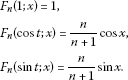We observe that the sequence $\left({L}_{n}\right)$ satisfies the conditions (2.2), (2.3) and (2.4). Hence, by Theorem 2.1, we have

${C}_{1}\left(st\right)\text{-}\underset{n\to \mathrm{\infty }}{lim}{\parallel {L}_{n}\left(f\right)-f\parallel }_{2\pi }=0,$

i.e. our theorem holds. But on the other hand, Theorem II does not hold for our operator defined by (4.1), since the sequence $\left({L}_{n}\right)$ is not convergent.

Hence, our Theorem 2.1 is stronger than that of Theorem II.

## References

1. Fast H: Sur la convergence statistique. Colloq. Math. 1951, 2: 241–244.

2. Steinhaus H: Sur la convergence ordinaire et la convergence asymptotique. Colloq. Math. 1951, 2: 73–74.

3. Móricz F:Tauberian conditions under whnih statistical corvergence follows fron statistical summability$\left(C,1\right)$. J. Math. Anal. Appl. 2002, 275: 277–287. 10.1016/S0022-247X(02)00338-4

4. Mohiuddine SA, Aiyub M: Lacunary statistical convergence in random 2-normed spaces. Appl. Math. Inform. Sci. 2012, 6(3):581–585.

5. Mohiuddine SA, Alghamdi MA: Statistical summability through a lacunary sequence in locally solid Riesz spaces. J. Inequal. Appl. 2012, 2012: 225. 10.1186/1029-242X-2012-225

6. Mohuiddine SA, Alotaibi A, Alsulami SM: Ideal convergence of double sequences in random 2-normed spaces. Adv. Differ. Equ. 2012, 2012: 149. 10.1186/1687-1847-2012-149

7. Mohiuddine SA, Alotaibi A, Mursaleen M: Statistical convergence of double sequences in locally solid Riesz spaces. Abstr. Appl. Anal. 2012., 2012: Article ID 719729

8. Mohiuddine SA, Lohani QMD: On generalized statistical convergence in intuitionistic fuzzy normed space. Chaos Solitons Fractals 2009, 42: 1731–1737. 10.1016/j.chaos.2009.03.086

9. Mohiuddine SA, Savaş E: Lacunary statistically convergent double sequences in probabilistic normed spaces. Ann. Univ. Ferrara, Sez. 7: Sci. Mat. 2012, 58: 331–339. 10.1007/s11565-012-0157-5

10. Mohiuddine SA, Şevli H, Cancan M: Statistical convergence in fuzzy 2-normed space. J. Comput. Anal. Appl. 2010, 12(4):787–798.

11. Mursaleen M, Mohiuddine SA: Statistical convergence of double sequences in intuitionistic fuzzy normed spaces. Chaos Solitons Fractals 2009, 41: 2414–2421. 10.1016/j.chaos.2008.09.018

12. Mursaleen M, Mohiuddine SA: On lacunary statistical convergence with respect to the intuitionistic fuzzy normed space. J. Comput. Appl. Math. 2009, 233: 142–149. 10.1016/j.cam.2009.07.005

13. Mursaleen M, Mohiuddine SA: On ideal convergence of double sequences in probabilistic normed spaces. Mathem. Rep. (Buchar.) 2010, 12(62)(4):359–371.

14. Mursaleen M, Mohiuddine SA: On ideal convergence in probabilistic normed spaces. Math. Slovaca 2012, 62: 49–62. 10.2478/s12175-011-0071-9

15. Mursaleen M, Mohiuddine SA, Edely OHH: On the ideal convergence of double sequences in intuitionistic fuzzy normed spaces. Comput. Math. Appl. 2010, 59: 603–611. 10.1016/j.camwa.2009.11.002

16. Savaş E, Mohiuddine SA:-statistically convergent double sequences in probabilistic normed spaces. Math. Slovaca 2012, 62(1):99–108. 10.2478/s12175-011-0075-5

17. Mursaleen M, Çakan C, Mohiuddine SA, Savaş E: Generalized statistical convergence and statistical core of double sequences. Acta Math. Sin. Engl. Ser. 2010, 26: 2131–2144. 10.1007/s10114-010-9050-2

18. Korovkin PP: Convergence of linear positive operators in the spaces of continuous functions. Dokl. Akad. Nauk SSSR 1953, 90: 961–964. (in Russian)

19. Korovkin PP: Linear Operators and Approximation Theory. Hindustan Publ. Co., Delhi; 1960.

20. Altomare F: Korovkin-type theorems and approximation by positive linear operators. Surv. Approx. Theory 2010, 5: 92–164.

21. Duman O: Statistical approximation for periodic functions. Demonstr. Math. 2003, 36: 873–878.

22. Demirci K, Dirik F: Approximation for periodic functions via statistical σ -convergence. Math. Commun. 2011, 16: 77–84.

23. Mursaleen M, Edely OHH: On invariant mean and statistical convergence. Appl. Math. Lett. 2009, 22: 1700–1704. 10.1016/j.aml.2009.06.005

24. Anastassiou GA, Mursaleen M, Mohiuddine SA: Some approximation theorems for functions of two variables through almost convergence of double sequences. J. Comput. Anal. Appl. 2011, 13(1):37–46.

25. Belen C, Mohiuddine SA: Generalized weighted statistical convergence and application. Appl. Math. Comput. 2013. doi:10.1016/j.amc.2013.03.115

26. Edely OHH, Mohiuddine SA, Noman AK: Korovkin type approximation theorems obtained through generalized statistical convergence. Appl. Math. Lett. 2010, 23: 1382–1387. 10.1016/j.aml.2010.07.004

27. Mohiuddine SA: An application of almost convergence in approximation theorems. Appl. Math. Lett. 2011, 24: 1856–1860. 10.1016/j.aml.2011.05.006

28. Mohiuddine SA, Alotaibi A: Statistical convergence and approximation theorems for functions of two variables. J. Comput. Anal. Appl. 2013, 15(2):218–223.

29. Mohiuddine SA, Alotaibi A, Mursaleen M:Statistical summability $\left(C,1\right)$and a Korovkin type approximation theorem. J. Inequal. Appl. 2012., 2012: Article ID 172

30. Mursaleen M, Alotaibi A: Statistical summability and approximation by de la Vallée-Poussin mean. Appl. Math. Lett. 2011, 24: 320–324. 10.1016/j.aml.2010.10.014

31. Mursaleen M, Alotaibi A: Statistical lacunary summability and a Korovkin type approximation theorem. Ann. Univ. Ferrara 2011, 57(2):373–381. 10.1007/s11565-011-0122-8

32. Mursaleen M, Karakaya V, Ertürk M, Gürsoy F: Weighted statistical convergence and its application to Korovkin type approximation theorem. Appl. Math. Comput. 2012, 218: 9132–9137. 10.1016/j.amc.2012.02.068

33. Srivastava HM, Mursaleen M, Khan A: Generalized equi-statistical convergence of positive linear operators and associated approximation theorems. Math. Comput. Model. 2012, 55: 2040–2051. 10.1016/j.mcm.2011.12.011

## Acknowledgements

This project was funded by the Deanship of Scientific Research (DSR), King Abdulaziz University, Jeddah, under grant no. (410/130/1432). The authors, therefore, acknowledge with thanks DSR technical and financial support.

## Author information

Authors

### Corresponding author

Correspondence to Abdullah Alotaibi.

### Competing interests

The authors declare that they have no competing interests.

### Authors’ contributions

The authors contributed equally and significantly in writing this paper. Both the authors read and approved the final manuscript.

## Rights and permissions

Reprints and Permissions

Mohiuddine, S.A., Alotaibi, A. Korovkin second theorem via statistical summability $\left(C,1\right)$. J Inequal Appl 2013, 149 (2013). https://doi.org/10.1186/1029-242X-2013-149

• Accepted:

• Published:

• DOI: https://doi.org/10.1186/1029-242X-2013-149

### Keywords

• density
• statistical convergence
• statistical summability $\left(C,1\right)$
• positive linear operator
• Korovkin type approximation theorem